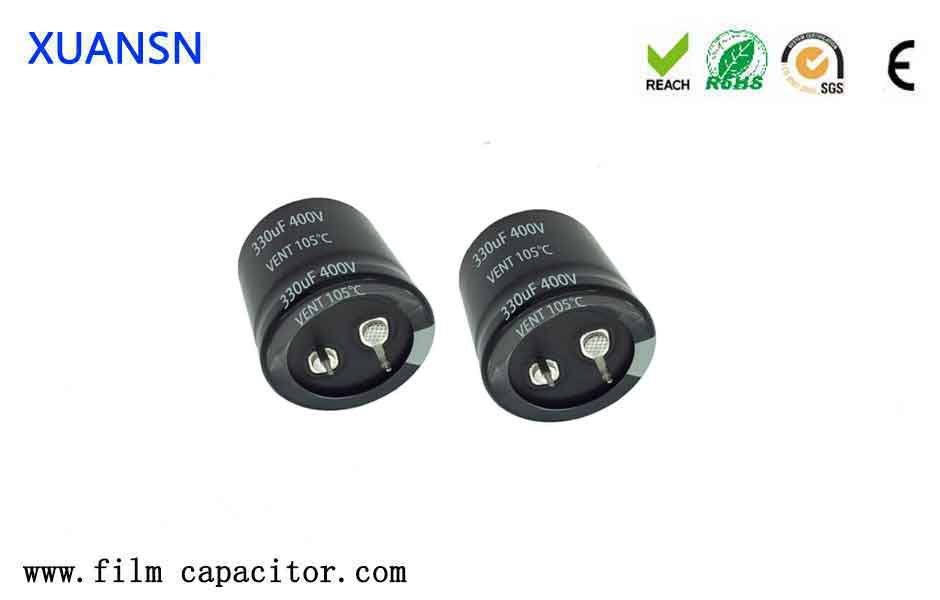Is the larger the capacitance the better?

Intuitively, it seems that the larger the energy storage capacitor, the stronger the current compensation capability provided by the IC. Therefore, many people like to use large-capacity capacitors. In fact, this is a wrong concept. Due to the existence of parasitic inductance on the capacitor, the capacitor discharge circuit will resonate at a certain frequency. At the resonance point, the impedance of the capacitor is small, so the impedance of the discharge circuit is the smallest, and the effect of supplementing energy is the best.But when the frequency exceeds the resonance point, the impedance of the discharge circuit begins to increase, which means that the capacity of the capacitor to provide current begins to decrease. The larger the capacitance of the capacitor, the lower the resonance frequency, and the smaller the frequency range in which the capacitor can effectively compensate for the current.

Therefore, in order to ensure the ability of the capacitor to provide high-frequency current, the larger the capacitor, the better. The larger the capacitance of the capacitor, the greater the amount of charge the capacitor can carry. Assuming that we regard the capacitor as a battery, every time the capacitor is charged and discharged, it can bring a greater load.

It is true that large-capacity capacitors can bring larger loads, but subsequently, the time for capacitor charging and discharging will increase, thereby reducing the high-frequency performance of the capacitor, and large capacitors often have greater parasitic inductance. This reduces the filtering effect and affects the stability of the circuit. Therefore, the capacity of the capacitor must be allocated according to the needs in order to achieve the best performance of the electrical appliance.

The use of a capacitor does not necessarily mean that a large capacity is good. It mainly depends on where it is used. The large capacity is large capacity, and the small capacity is small capacity. Appropriateness is important.Is the larger the capacitance the better?